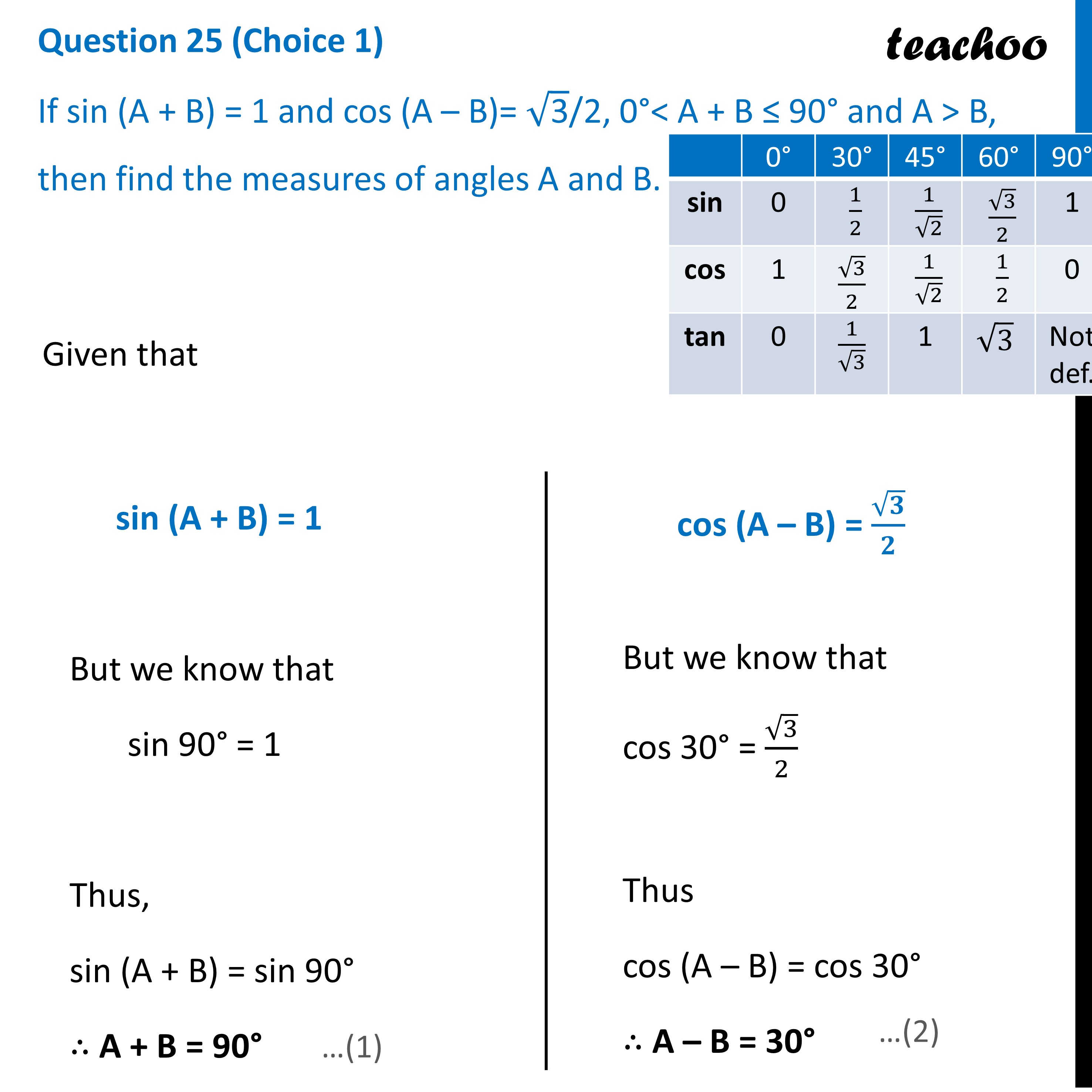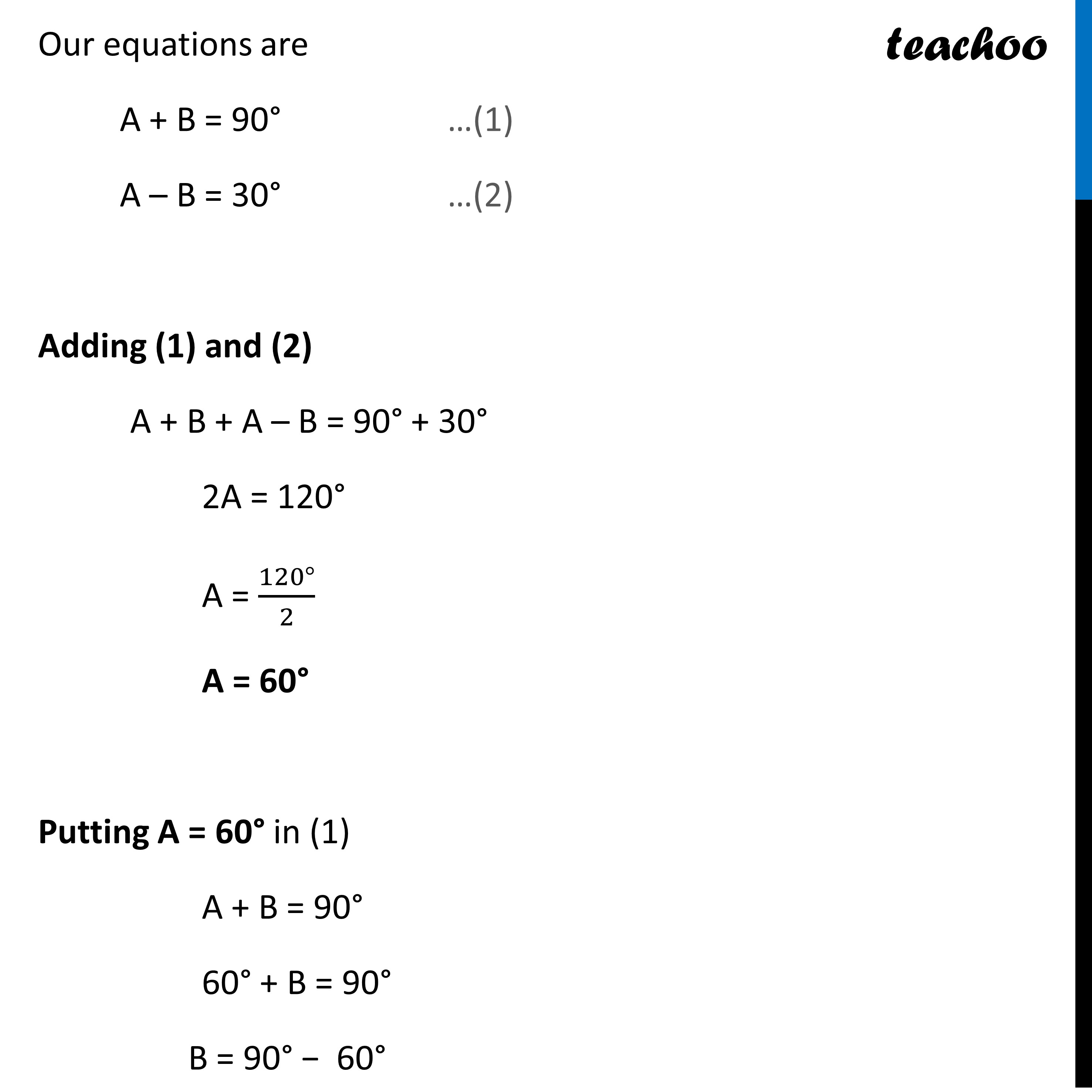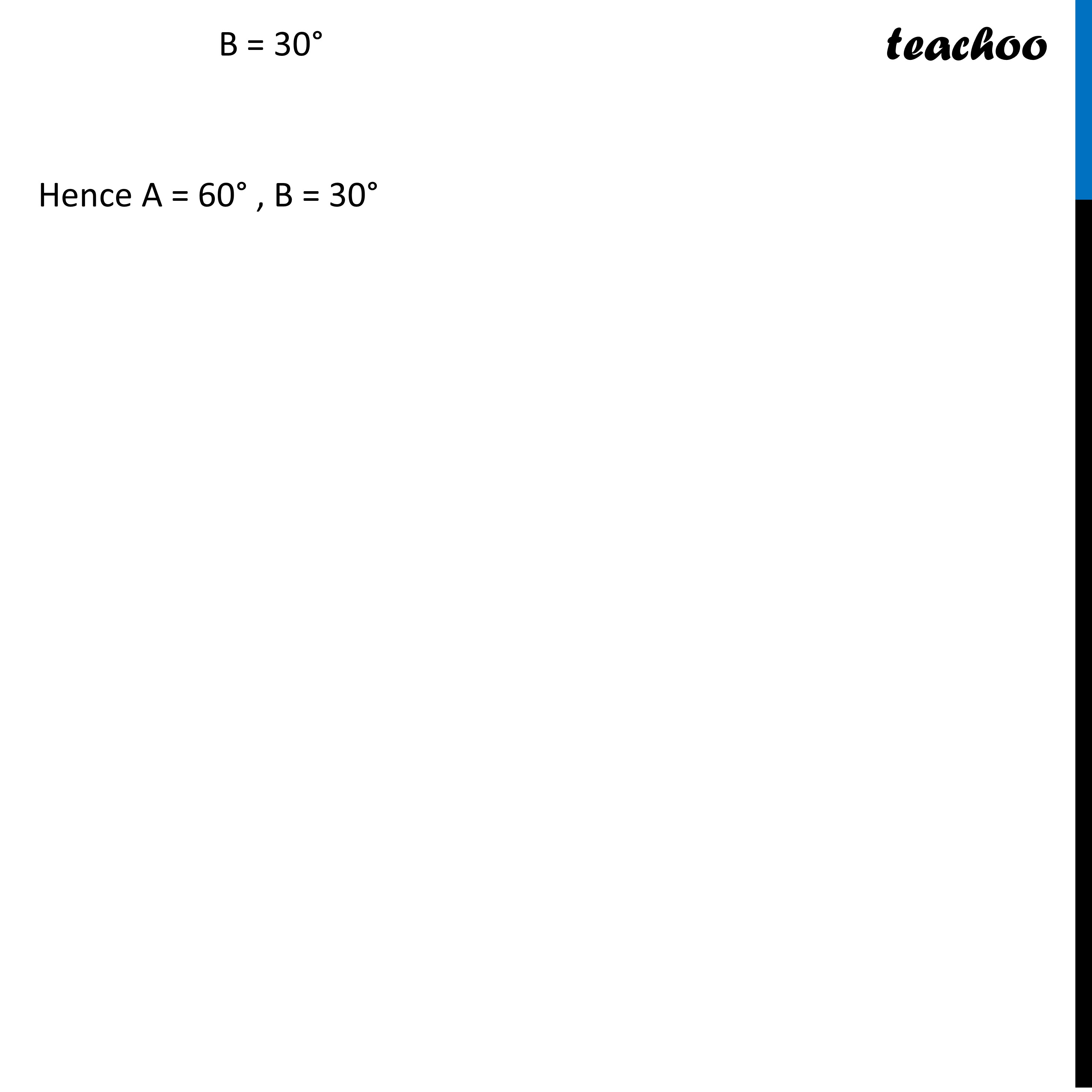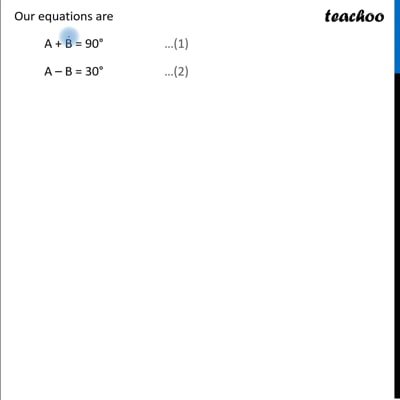CBSE Class 10 Sample Paper for 2023 Boards - Maths Standard

Class 10
Solutions of Sample Papers for Class 10 Boards

## If sin(A+B) = 1 and cos (A - B)= √3/2, 0°< A+B ≤ 90° and A> B, then find the measures of angles A and B.

This question is Similar to Ex 8.2,3 - Chapter 8 Class 10This video is only available for Teachoo black users

Get live Maths 1-on-1 Classs - Class 6 to 12

### Transcript

Question 25 (Choice 1) If sin (A + B) = 1 and cos (A – B)= √("3" )/2, 0°< A + B ≤ 90° and A > B, then find the measures of angles A and B.Given that sin (A + B) = 1 But we know that sin 90° = 1 Thus, sin (A + B) = sin 90° ∴ A + B = 90° cos (A – B) = √𝟑/𝟐 But we know that cos 30° = √3/2 Thus cos (A – B) = cos 30° ∴ A – B = 30° Our equations are A + B = 90° …(1) A – B = 30° …(2) Adding (1) and (2) A + B + A – B = 90° + 30° 2A = 120° A = (120°)/2 A = 60° Putting A = 60° in (1) A + B = 90° 60° + B = 90° B = 90° − 60° B = 30° Hence A = 60° , B = 30°